# MR Second Printing

## Contents

### Overall

There are a few places in the book where we have an italic T instead of a roman T for transpose, often where they are preceded by a negative (inverse) sign. These should be corrected. The ones we're aware of are listed below:

• Equation (5.4) p149
• Fifth line of p163
• p168 third line of Eq (5.27)
• Last line of p216
• p239: eq (8.21) and equation just above.
• p254: Eqs (8.75), (8.76), (8.78), (8.79), 8.80)
• pp256-7: several instances in Ch 8.6. Just after eq (8.87) (two times), four times in eq (8.88), just after (8.88), and (8.90) and (8.91).
• p272, in each of the two displayed equations in the 2nd bullet
• p491, second-to-last bullet, in each of the two displayed equations and the in-line equation just after.

### Chapter 1

• p3, middle: Change "convenient way to calculate a lower bound on the dof" to "convenient way to calculate the dof"

### Chapter 2

• Proposition 2.2, p16: "This formula holds only if all joint constraints are independent. If they are not independent then the formula provides a lower bound on the number of degrees of freedom." should be "This formula holds in "generic" cases, but it fails under certain configurations of the links and joints, such as when the joint constraints are not independent."
• Chapter 2.2.2, Example 2.3, p16: "Substituting" is misspelled.
• Eliminate two sentences on p19: "Grubler's formula provides ... are discussed in Chapter 7."
• Fifth bullet of Chapter 2.6 p32: Eliminate the last sentence "If the constraints enforced ... degrees of freedom."

### Chapter 3

• In the caption of Figure 3.3 p53,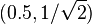$(0.5,1/\sqrt{2})$ should read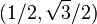$(1/2, \sqrt{3}/2)$, and$(-1/\sqrt{2},0.5)$ should read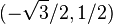$(-\sqrt{3}/2, 1/2)$.
• Under "Representing a configuration" in Chapter 3.3.1.2 p78 near the bottom, the text says "Tbc = (Rbc,pbc) represents {b} relative to {c}" but it should say "represents {c} relative to {b}".
• There is a typo in the final matrix, Tce, of Example 3.19, p82. The term$130/\sqrt{2}$ should be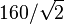$160/\sqrt{2}$.
• In the displayed equation just after Equation (3.76) p85, the left-hand side should be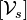$[\mathcal{V}_s]$ (the brackets are missing).
• Near the end of Chapter 3.6 Software p97, the function "AxisAng" should be written "AxisAng6."
• Exercise 3.16(i) p103 asks for "the"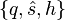$\{q,\hat{s},h\}$ representation but it should say "a"$\{q,\hat{s},h\}$ representation (since q is not unique). Replace "the" with "a".

### Chapter 4

• Example 4.1, last row of the table (screw axis for joint 3), middle of p122: The linear component vi should be (0, − L2,0) (the sign is missing).

### Chapter 5

• Equation (5.7) p153: The the first two terms on the right-hand side of the equation should be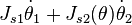$J_{s1} \dot{\theta}_1 + J_{s2}(\theta) \dot{\theta}_2$.
• Exercise 5.2(b) p173: Change (a) to "Suppose that the last link must apply a wrench corresponding to a force of ..." (then continue as current, "5 N in the..."). In (b), change "the tip" to "the last link".
• Figure 5.15(b) p174: The circular arrow indicating the rotation of joint 3 is slightly misplaced.
• Exercise 5.11(a) p178: Change "What are the..." to "Is this motion possible? If so, what are the ..."
• Exercise 5.16 p180: change "PRRRRR" to "PRPRRR". Similarly, caption of Figure 5.26, p181, change "PRRRRR" to "PRPRRR."
• Exercise 5.25(b) p186: At the end of part (b), add the sentence "Comment on why it is usually preferred to use the body Jacobian instead of the space Jacobian for the manipulability ellipsoid."

### Chapter 6

• Chapter 6.3, first sentence after Eq (6.7), p199: the matrices$T_{sd}^{-1} \dot{T}_{sd}$ and$\dot{T}_{sd} T_{sd}^{-1}$ are referred to as twists, but these are the se(3) matrix representations of the twists. Change the beginning of this sentence to "The matrix form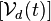$[\mathcal{V}_d(t)]$ of the desired twist is either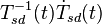$T_{sd}^{-1}(t) \dot{T}_{sd}(t)$ (the matrix form of the body twist of the desired trajectory at time t) or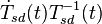$\dot{T}_{sd}(t) T_{sd}^{-1}(t)$ (the matrix form of the spatial twist), depending ..." and then complete the sentence as is.

### Chapter 7

• p218 displayed equation after (7.12): replace ϕ5 with ψ5 in qp to be consistent with definition of qa.
• Just below caption for Figure 7.8 p222: There is an extraneous dot.

### Chapter 8

• Description of constrained dynamics (see conversation with Zack).
• In Equation (8.14) p236,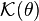$\mathcal{K}(\theta)$ should be replaced by$\mathcal{K}(\theta,\dot{\theta})$.
• Figure 8.5 p244 says the volume of the rectangular parallelepiped is abc but it should be hlw.
• End of first sentence after Eq (8.26) in Section 8.2.1 p244 should read "... columns of Rbc correspond to the eigenvectors of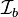$\mathcal{I}_b$." (The word "eigenvalues" should be replaced by "eigenvectors.")
• pp 249-50: We would like to clarify
From the fact $\dot{T}_{i,i-1} T_{i,i-1}^{-1}= -[\mathcal{A}_i \dot{\theta}_i]$, we have
$\dot{R}_{i,i-1} = -[\omega\dot{\theta}_i]R_{i,i-1}, \quad \dot{p} = -[\omega\dot{\theta}_i]p -v\dot{\theta}_i.$
Then
\begin{align*}
& = \frac{d}{dt}
\begin{bmatrix}
R_{i,i-1} && 0\\
[p]R_{i,i-1} && R_{i,i-1}
\end{bmatrix} \mathcal{V}_{i-1}\\
& = \begin{bmatrix}
-[\omega\dot{\theta}_i]R_{i,i-1} && 0\\
[-[\omega\dot{\theta}_i]p -v\dot{\theta}_i] R_{i,i-1}-[p][\omega\dot{\theta}_i]R_{i,i-1} && -[\omega\dot{\theta}_i]R_{i,i-1}
\end{bmatrix}
\mathcal{V}_{i-1}\\
& = \underbrace{\begin{bmatrix}
-[\omega\dot{\theta}_i] && 0\\
-[v\dot{\theta}_i] && -[\omega\dot{\theta}_i]
\underbrace{\begin{bmatrix}
R_{i,i-1} && 0\\
[p]R_{i,i-1} && R_{i,i-1}
\end{align*}
where the transition from the second equality to the third follows from the Jacobi identity $a \times (b \times c) + b \times (c \times a) + c \times (a \times b) = 0$ for all $a, b, c \in \real^3$, and the transition from the fourth equality to the fifth follows from the identity $[\operatorname{ad}_{V_1}] V_2 = - [\operatorname{ad}_{V_2}] V_1$.


• p258: In the parenthetical text a few lines before Equation (8.95): "...there is an equality constraint..." should instead be "...there is also an inequality constraint..."
• Caption of Figure 8.10 p264: The operating region is light gray and the continuous operating region is dark gray (swap the two parenthetical statements).
• Exercise 8.6(a) p276: The expression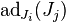$\text{ad}_{J_i}(J_j)$ has the indices switched; the correct expression is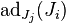$\text{ad}_{J_j}(J_i)$.
• Exercise 8.7 p276: The expression should be written: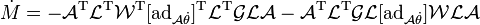$\dot{M} = -\mathcal{A}^{\rm T} \mathcal{L}^{\rm T} \mathcal{W}^{\rm T} [\mbox{ad}_{\mathcal{A} \dot{\theta}}]^{\rm T} \mathcal{L}^{\rm T} \mathcal{GLA} - \mathcal{A}^{\rm T} \mathcal{L}^{\rm T} \mathcal{GL} [\mbox{ad}_{\mathcal{A} \dot{\theta}}] \mathcal{WLA}$

### Chapter 10

• Equation (10.4) p332 should read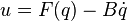$u = F(q) - B \dot{q}$ (the plus sign in the book should be a minus sign).
• p335, first line of chapter 10.6.4: should be$u = F(q) - B \dot{q}$ (plus sign should be a minus sign). In the second-to-last line on the same page, the plus sign should be a minus sign.

### Chapter 11

• In the displayed equation after Equation (11.18) p360, the vector Xe(t) is a six-vector. The bottom three elements are written correctly, but the top three elements, an angular velocity, are written instead in their 3x3 so(3) form. Also, the term written RT(d) should be written RT(t). So, replace the top three elements in the vector by ωe(t), make the period after the equation a comma, and insert a line immediately following saying "where e(t)] = log(RT(t)Rd(t))."
• In Equation (11.33) p366, the right-hand side should be zero, not c.
• Figure 11.24 p384, the Robonaut 2 series elastic actuator, fourth sentence of the caption: The words "outer" and "inner" should be switched, so the new sentence reads "The inner ring of hole mounts connects to the harmonic gearhead output, and the outer ring of hole mounts is the output of the SEA, connecting to the next link."

### Chapter 12

• In Example 12.4 p404, the elements of the wrenches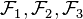$\mathcal{F}_1, \mathcal{F}_2, \mathcal{F}_3$ are erroneously written in the order (fx,fy,mz); they should be written in the order (mz,fx,fy). In other words, change these to ( − 2,0,1), (1, − 1,0), and (1,1,0), respectively.
• Caption of Figure 12.14 p412, second-to-last sentence: "rotation if possible" should be "rotation is possible."

### Chapter 13

• Equation (13.29) p467: yp should be yP (P is capitalized).
• p469, first line just below the figure: get rid of the absolute value signs, so should be $v_d \neq 0$.

### Appendix C

• Section C.3, Equation (C.5) p507: All four instances of ϕi − 1 should be replaced by ϕi.
• In Equation (C.12) p510, two instances of Mi − 1 should be replaced by Mi.

### A partial list of errata contributors

Thanks to the following people who provided corrections, starting from the preliminary version of the book posted in October, 2016:

H. Andy Nam, Eric Lee, Yuchen Rao, Chainatee Tanakulrongson, Mengjiao Hong, Kevin Cheng, Jens Lundell, Elton Cheng, Michael Young, Jarvis Schultz, Logan Springgate, Sofya Akhmametyeva, Aykut Onol, Josh Holcomb, Yue Chen, Mark Shi, AJ Ibraheem, Yalun Wen, Seongjae Jeong, Josh Mehling, Felix Wang, Drew Warren, Chris Miller, Clemens Eppner, Zack Woodruff, Jian Shi, Jixiang Zhang, Shachar Liberman, Will Wu, Dirk Boysen, Awe Wang, Ville Kyrki, John Troll, Andrew Taylor, Nikhil Bakshi